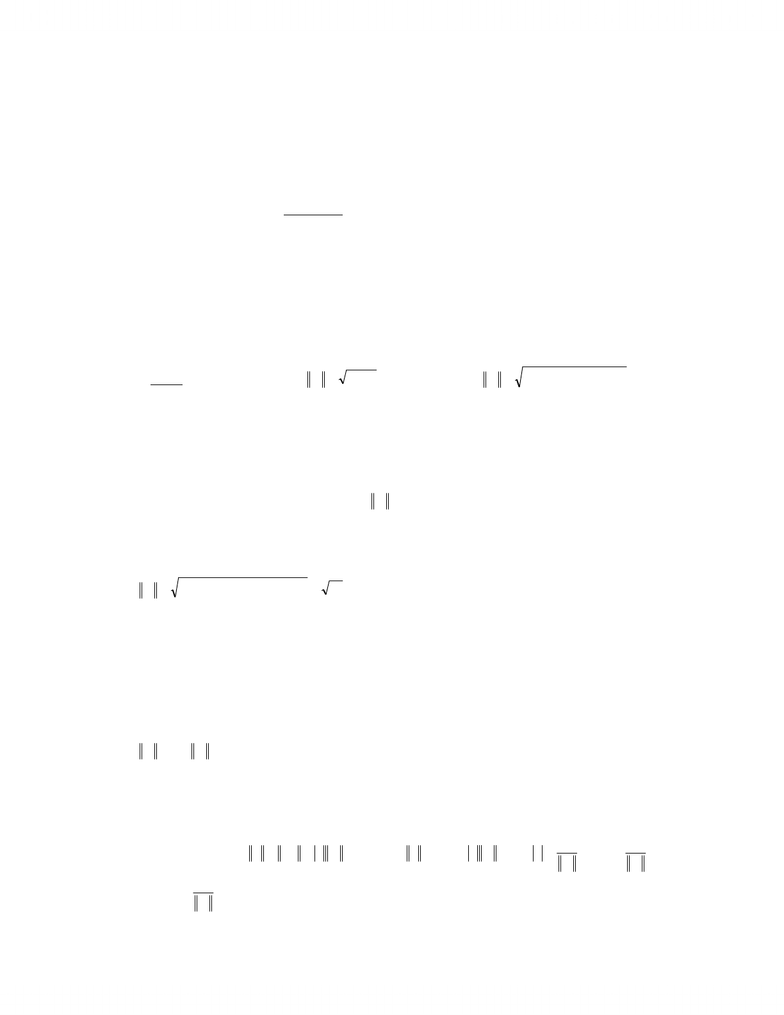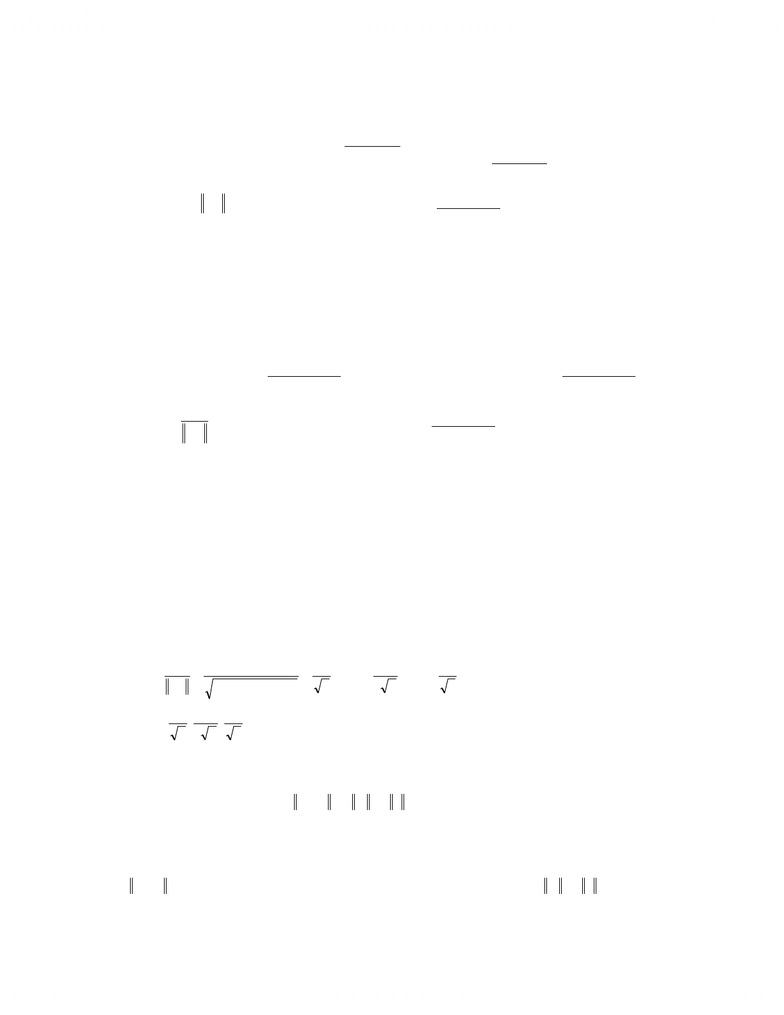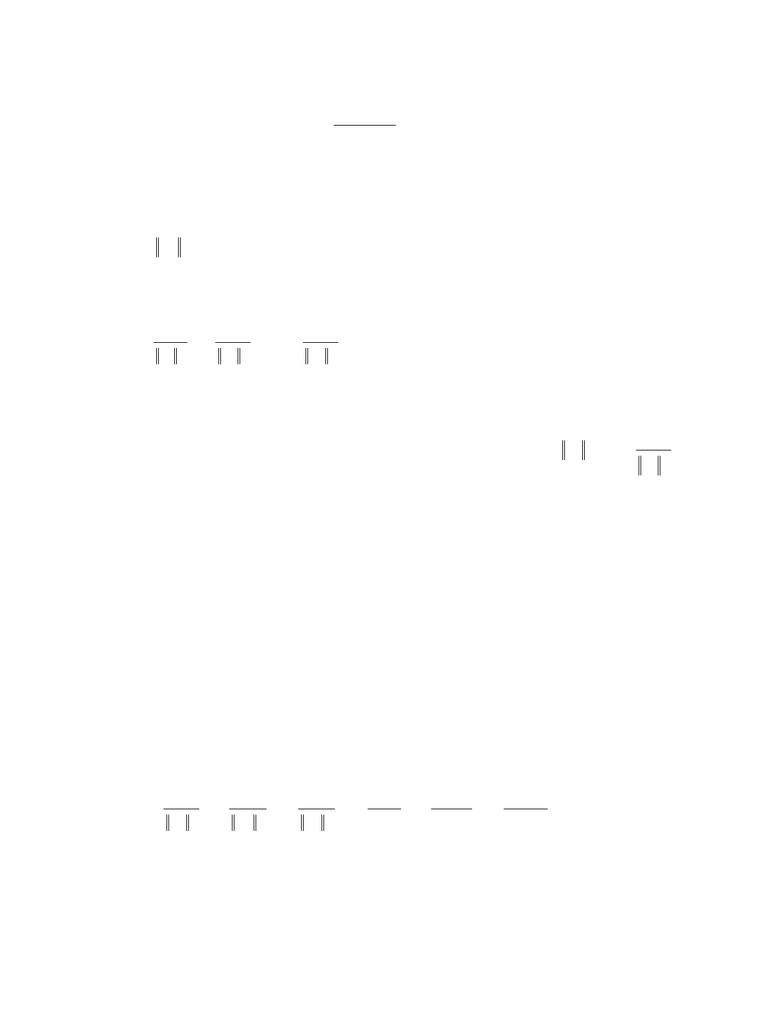# Summary notes 2End

52 views51 pagesMAT223H1b.doc
Page 1 of 51
Lecture #11 Tuesday, February 10, 2004
ORTHOGONALITY
Definition
Let X, Y be vectors in Rn. The dot product is
R
=
Y
X
Y
X
T.
So, if
=
n
x
x
x
X
2
1
,
=
n
y
y
y
Y
2
1
,
=
=+++=
n
j
jjnn yxyxyxyxYX
1
2211 .
Definition
The length of a vector X, denoted XXX =. So if
=
n
x
x
x
X
2
1
, 0
22
2
2
1+++= n
xxxX.
Example
Let
=
4
2
0
1
X,
=
2
3
2
1
Y. Find
Y
X
and X.
(
)
(
)
(
)
(
)
(
)
(
)
(
)
(
)
1524322011
=
+
+
+
=
YX.
( ) ( ) ( ) ( )
214201 2222 =+++=X.
Theorem
Let X, Y, Z be vectors in Rn. Then:
1)
X
Y
Y
X
=
. (Proof:
==
=
n
j
jj
n
j
jj xyyx
11
)
2)
(
)
ZXYXZYX
+
=
+
.
3) For a R,
(
)
(
)
(
)
YXaaYXYaX
=
=
.
4) 0X, 00 ==xX.
Example
Let X be a vector in Rn, X 0. Find all Y Rn such that Y is collinear to X and is unitary.
Let
R
=
aaXY,. XaaXY== . We want 1=Y, so X
a
X
aXa11
1±===.
So, X
X
Y1
±= .
www.notesolution.com
Unlock document

This preview shows pages 1-3 of the document.
Unlock all 51 pages and 3 million more documents.MAT223H1b.doc
Page 2 of 51
Definition
Let X, Y be two vectors in Rn. X and Y are orthogonal iff 0
=
YX.
Let
k
XXX ,,, 21 be a set of vectors in Rn.
k
XXX ,,, 21 is orthogonal iff kixi,,2,1,0
=
and jiXX ji = ,0.
Moreover, if kjXj,,2,1,1== , then {X1, X2,, Xk} is orthonormal.
Example
The standard basis of Rn is a orthonormal set.
=
0
0
1
1
E,
=
0
1
0
2
E,
=
1
0
0
3
E.
Definition
If
k
XXX ,,, 21 is an orthogonal set, then
kk XaXaXa,,, 2211 is also an orthogonal set if
kjaj,,2,1,0
=
.
For
j
jX
a1
=, the set
kk XaXaXa,,, 2211 will be orthonormal.
Example
Let
=
1
1
2
1
X,
=
3
3
0
2
X,
=
1
1
1
3
X. Construct an orthonormal set.
321 ,, XXX is an orthogonal set because:
0
i
X.
0
21
=
XX , 0
31
=
XX , 0
32
=
XX .
Find 3,2,1,
=
jaj such that
332211 ,, XaXaXa is orthonormal:
( ) ( ) ( )
6
1
112
11
222
1
1=
++
== X
a,
23
1
2=a,
3
1
3=a.
So,
3
,
23
,
6
3
21 X
XX is orthonormal.
Theorem (Pythagorean)
If X and Y are orthogonal, then 222 YXYX+=+ .
Proof
X and Y are orthogonal; it means 0
=
YX. So,
( ) ( )
222 00 YXYYXXYYXYYXXXYXYXYX+=+++=+++=++=+ .
www.notesolution.com
Unlock document

This preview shows pages 1-3 of the document.
Unlock all 51 pages and 3 million more documents.MAT223H1b.doc
Page 3 of 51
Theorem
Every orthogonal set of vectors of Rn is independent.
Proof
Let
k
XXX ,,, 21 be an orthogonal set. Consider 0
2211
=
+
+
+
=
kk XtXtXtY. We want to prove
that 0
=
j
t.
(
)
00
00
2
22112211
==
=
+
+
+
+
+
=
+
+
+
=
jjj
kkjjjjjkkjj
tXt
XtXXtXXtXXtXtXtXtXYX
.
Theorem
If
n
EEE ,,, 21 is an orthogonal basis of Rn, then n
X
R
,
n
n
nE
E
EX
E
E
EX
E
E
EX
X2
2
2
2
2
1
2
1
1
++
+
=.
Proof
Let X Rn. Then nn EtEtEtX
+
+
+
=
2211 because is a
n
EEE ,,, 21 basis of Rn.
( )
2
2
112211
j
j
jjjjnnjjjjjnnj
E
EX
tEtEEtEEtEEtEEtEtEtEX
==++++=++=.
Example
Let
=
0
1
2
1
X,
=
3
3
0
2
X,
=
1
1
1
3
X. Show that
321 ,, XXX is an orthogonal basis of R3.
It is enough to prove 0
21
=
XX , 0
32
=
XX , 0
31
=
XX because:
321 ,, XXX is an orthogonal set, so X1, X2, X3 are independent.
3dim3=R. So a set of 3 linearly independent vectors is a basis.
Example
Write
=
c
b
a
X as a linear combination of
=
0
1
2
1
X,
=
3
3
0
2
X,
=
1
1
1
3
X.
Since
321 ,, XXX is orthogonal,
3213
2
3
3
2
2
2
2
1
2
1
1
36
3
5
2X
cba
X
cb
X
ba
X
X
XX
X
X
XX
X
X
XX
X+
+
+
+
+
=
+
+
=.
www.notesolution.com
Unlock document

This preview shows pages 1-3 of the document.
Unlock all 51 pages and 3 million more documents.